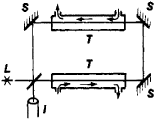# Fizeau Experiment

Also found in: Wikipedia.

## Fizeau Experiment

an experiment for determining the speed of light in moving media or bodies. The Fizeau experiment was carried out by A.-H.-L. Fizeau in 1851 and showed that light is partially dragged along by a moving medium. The speed of light in a moving medium is equal to c/n ± αv, where c/n is the speed of light in a stationary medium (c is the speed of light in a vacuum, and n is the refractive index of the medium), v is the velocity of the medium relative to an observer (that is, the velocity in the laboratory reference frame), α is the drag coefficient, and the signs + and – correspond to the cases where the light and the medium travel in the same direction and in opposite directions, respectively. The Fizeau experiment confirmed the equation previously obtained by A. Fresnel for the drag coefficient: α = 1 – 1/n2.

A schematic diagram of the Fizeau experiment is presented in Figure 1. A light beam from a source L is divided by a semitransparent plate into two beams. One of the beams, after being reflected from mirrors S, passes through water flowing in tubes T; this beam travels in the same direction as the water flow, but the second beam travels in the opposite direction. Both beams are directed to an interferometer I, and an interference pattern is observed. Measurements are made first in stationary water and then in moving water. The difference between the times required for the beams to travel in the moving water and, consequently, the coefficient a are determined from the shift of the interference fringes.Figure 1

The Fizeau experiment played an important part in the development of the electrodynamics of moving media and was later an experimental proof of A. Einstein’s special theory of relativity, in which α is obtained directly from the relativistic equation for addition of velocities if terms higher than first order in v/c are neglected (seeRELATIVITY, THEORY OF). Allowance for dispersion, that is, for the dependence of n on the wavelength λ. of light, yields the term ∓(λ/n)(∂n/∂λ)v in the drag coefficient. The term was obtained theoretically by H. Lorentz and was experimentally verified in 1914 by P. Zeeman and co-workers.

K. I. POGORELOV [27–1132–3 ]

References in periodicals archive ?
This effect is explained by the Fresnel expression in (1), which was also confirmed by the Fizeau experiment in 1851, who used two beams of light travelling through two tubes filled with flowing water, with one beam flowing in the direction of the water, and the other counterflowing.

Site: Follow: Share:
Open / Close